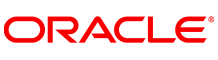New update is available. Click here to update.
Topics

# Reverse Nodes in k-Group

Hard0/120
Average time to solve is 56m+15 more companies

## Problem statement

You are given a Singly Linked List of integers and an integer array 'B' of size 'N'. Each element in the array 'B' represents a block size. Modify the linked list by reversing the nodes in each block whose sizes are given by the array 'B'.

Note:
``````1. If you encounter a situation when 'B[i]' is greater than the number of remaining nodes in the list, then simply reverse the remaining nodes as a block and ignore all the block sizes from 'B[i]'.

2. All block sizes are contiguous i.e. suppose that block 'B[i]' ends at a node cur, then the block 'B[i+1]' starts from the node just after the node cur.
``````
Example
``````Linked list: 1->2->3->4->5
Array B: 3 3 5

Output: 3->2->1->5->4

We reverse the first block of size 3 and then move to block 2. Now, since the number of nodes remaining in the list (2) is less than the block size (3), we reverse the remaining nodes (4 and 5) as a block and ignore all the block sizes that follow.
``````
Detailed explanation ( Input/output format, Notes, Images )
Constraints:
``````0 <= L <= 5 * 10^5
-10^9 <= data <= 10^9 and data != -1
1 <= N <= 5 * 10^5
0 <= B[i] <= 5 * 10^5

Where 'L' is the number of nodes in the linked list and 'data' is the value of a node in the linked list.

Time Limit: 1 sec
``````
Sample Input 1:
``````1 2 3 4 5 6 7 8 9 10 11 -1
3
2 3 4
``````
Sample Output 1:
``````2 1 5 4 3 9 8 7 6 10 11 -1
``````
Explanation of the Sample Output 1:
``````For the given input, the block sizes are 2, 3 and 4 respectively. First, we reverse 2 elements (1->2 becomes 2->1), then the next 3 elements (3->4->5 becomes 5->4->3) and lastly the next 4 elements (6->7->8->9 becomes 9->8->7->6). Thus, the final modified list becomes 2->1->5->4->3->9->8->7->6->10->11.
``````
Sample Input 2:
``````0 6 1 5 -1
2
2 3
``````
Sample Output 2:
``````6 0 5 1 -1
``````
Explanation of the Sample Output 2:
``````For the given input, the block sizes are 2 and 3 . First, we reverse 2 elements (0->6 becomes 6->0), then we need to change next 3 elements but we are left with only 2 elements (1->5) and thus it becomes (5->1). Thus, the final modified list becomes 6->0->5->1.
``````Console